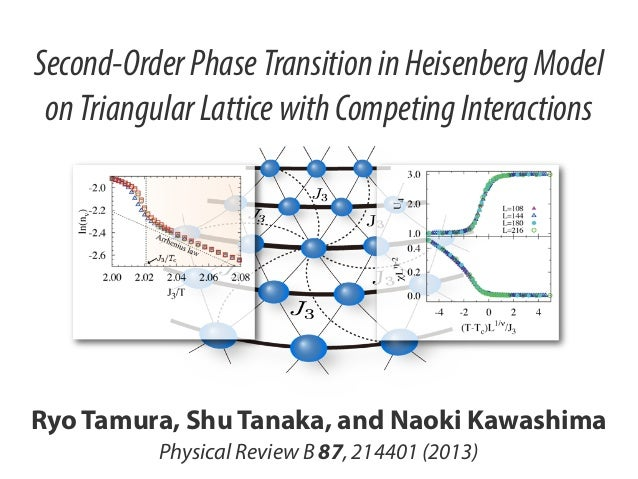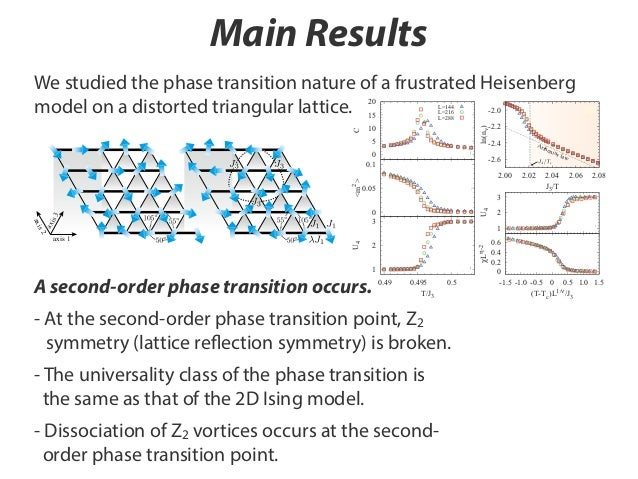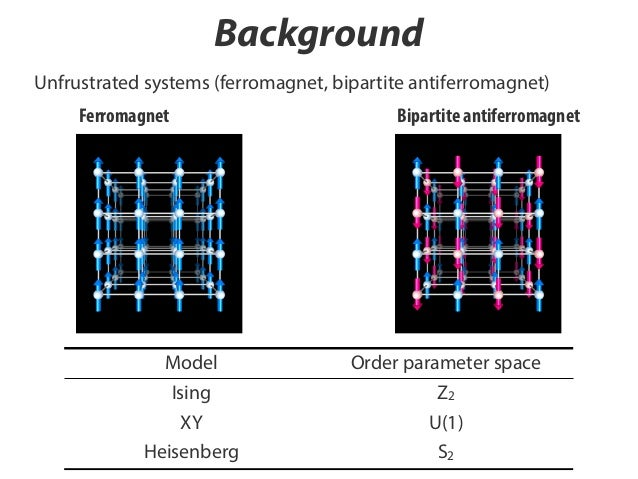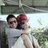Successfully reported this slideshow.Upcoming SlideShare
×

ofUpcoming SlideShare
２次元可解量子系のエンタングルメント特性
Next

1 Like

Share

# Second-Order Phase Transition in Heisenberg Model on Triangular Lattice with Competing Interactions

Our paper entitled “Second-Order Phase Transition in Heisenberg Model on Triangular Lattice with Competing Interactions" was published in Physical Review B. This work was done in collaboration with Dr. Ryo Tamura (NIMS) and Professor Naoki Kawashima (ISSP).
http://prb.aps.org/abstract/PRB/v87/i21/e214401

NIMSの田村亮さん、物性研の川島直輝教授との共同研究論文 “Second-Order Phase Transition in Heisenberg Model on Triangular Lattice with Competing Interactions" が Physical Review B に掲載されました。
http://prb.aps.org/abstract/PRB/v87/i21/e214401

See all

See all

### Second-Order Phase Transition in Heisenberg Model on Triangular Lattice with Competing Interactions

1. 1. Second-Order Phase Transition in Heisenberg Model on Triangular Lattice with Competing Interactions Ryo Tamura, Shu Tanaka, and Naoki Kawashima Physical Review B 87, 214401 (2013)
2. 2. Main Results SECOND-ORDER PHASE TRANSITION IN THE . . . We studied the phase transition nature of a frustrated Heisenberg model on a distorted triangular lattice. 20 L=144 L=216 L=288 -2.2 ln(nv) 5 (a) 2 <m > 0 0.1 (b) Arr hen ius -2.6 2.00 2.02 0.05 s2 U4 U4 axi s3 2.06 2.08 0 2 (e) 1 2 (c) 1 A second-order phase transition occurs. 2.04 J3/T law 3 0 3 axi axis 1 0 -2.4 Tc/J3 10 0.49 0.495 T/J3 0.5 χLη-2 C 15 0 (d) -2.0 (f) 0.6 0.4 0.2 0 -1.5 -1.0 -0.5 0 0.5 1.0 1.5 (T-T c)L1/ν/J3 FIG. (Color - At the second-order phase transition physical 2.quantitiesonline) Temperature Jdependence offor J /J = point, Z2 of the distorted -J model equilibrium −0.4926 . . and λ = 1.308 . (a) Speciﬁc (b) Square of symmetry (lattice reflection symmetry)order.broken.. (c). ..Binder ratio Uheat C.Log of number is parameter m . (d) the 1 2 3 1 3 4 density of Z2 vortex nv versus J3 /T . The dotted vertical line indicates the transition temperature Tc /J3 = 0.4950(5). (e) and (f) Finite-size scaling of the Binder ratio U4 and that of the susceptibility χ using the critical exponents of the 2D Ising model (ν = 1 and η = 1/4) and the transition temperature. Error bars are omitted for clarity since their sizes are smaller than the symbol sizes. - The universality class of the phase transition is the same as that of the 2D Ising model. - Dissociation of Z2 vortices occurs at the secondIn antiferromagnetic Heisenberg models on a triangular lattice, the dissociation of the Z vortices occurs at ﬁnite order phase transition point. temperature. 13,27 2 In order to conﬁrm the dissociation of the mod ope ﬁrst soli pha size λ= the are of t vie ﬁgu smo and wh sca λ=
3. 3. Background Unfrustrated systems (ferromagnet, bipartite antiferromagnet) Ferromagnet Bipartite antiferromagnet Model Ising Order parameter space Z2 XY Heisenberg U(1) S2
4. 4. Background Frustrated systems Antiferromagnetic Ising model on triangle Antiferromagnetic XY/Heisenberg model on triangle ? triangular lattice kagome lattice pyrochlore lattice
5. 5. Background Order parameter space in antiferromagnet on triangular lattice. Model Ising XY Order parameter space --U(1) Phase transition --KT transition Heisenberg SO(3) Z2 vortex dissociation
6. 6. Motivation To investigate the finite-temperature properties in two-dimensional systems whose order parameter space is SO(3)xZ2. - Phase transition occurs? - Z2 vortex dissociation?
7. 7. Model H = J1 i,j axis 1 si · sj + J1 1st nearestneighbor axis 1 i,j axis 2,3 si · sj + J3 1st nearestneighbor axis 2, 3 i,j si · sj > 0, J3 > 0 3rd nearestneighbor s2 axi axi s3 si : Heisenberg spin (three components) axis 1
8. 8. Ground State Spiral-spin configuration si = R cos(k · ri ) I sin(k · ri ) R, I are two arbitrary orthogonal unit vectors. Fourier transform of interactions J(k) J1 2J1 kx = cos kx + cos cos N J3 J3 J3 2 3ky + cos 2kx + 2 cos kx cos 2 3ky Find k that minimizes the Fourier transform of interactions! 4 < J1 /J3 < 0
9. 9. SO(3) x C3 & SO(3) x Z2 (ii) single-k spiral (a) (c) structure sp ira l 4 independent sublattices )t rip lek structure (iv axis 3 axis 2 axis 1 (b) (iii) double-k spiral (ii) single-k spiral (i) ferromagnetic R. dotted hexagonal area in Soc. The Fig. 1. (a) Triangular lattice with L x × Ly sites. (b) Enlarged view of theTamura and N. Kawashima, J. Phys. (a). Jpn., 77, 103002 (2008). R. Tamura and N. Kawashima, J. i-th site are thick and thin lines indicate λJ1 and J1 , respectively. The third nearest-neighbor interactions at thePhys. Soc. Jpn., 80, 074008 (2011). R. Tamura and S. categorized into depicted. (c) Ground-state phase diagram of the model given by Eq. (1). Ground states can be Tanaka, Phys. Rev. E, 88, 052138 (2013). R. Tamura, S. Tanaka, and N. Kawashima, to appear in Proceedings of APPC12. ﬁve types. More details in each ground state are given in the main text. J1-J3 model on triangular lattice discussed the connection between frustrated continuous spin systems and a fundamental discrete spin Order parameter space Order of phase transition system by using a locally deﬁned parameter. The most famous example is the chiral phase transition in the antiferromagnetic XY model on a triangular lattice. The relation between the phase transition SO(3)xC3 that of the Ising model has been established [24, 25]. In this paper, 1st order of the continuous spin system and we study ﬁnite-temperature properties in the J1 -J3 model on a distorted triangular lattice depicted in SO(3)xZ2 2nd order (Ising universality) Figs. 1(a) and (b) from a viewpoint of the Potts model with invisible states.
10. 10. SECOND-ORDER PHASE TRANSITION IN THE . . . Specific heat, order parameter SECOND-ORDER PHASE TRANSITION IN THE . . . axi U4 0.495 2 T/J3 (t) 0.5 3 (d) 0.4 (t) 2.02 (t) s3 2.04 , m := J3/T (e) (f) 0.6 2 0.4 0.2 1 0 2 -1.5 -1.0 -0.5 0 0.5 1.0 1.5 0.6 (T-T c)L1/ν/J3 0.4 0.2 2.06 0 2 (t) /N 1 t U4 Phase transition with FIG. model fo (e) Z symmtery breaking open squ (f) ﬁrst-orde occurs. solid circ χL (c) (t) := s1 · s2 ( Tc/J3 ln(nv) C 2 <m > 0.49 U4 2 U4 s2 1 2.00 si0.6j ·s = 1.308 · · · rrhe 2.06 A2.08 niu s la w 2.04 J -2.6 3/T 3 (b) 0.4926 · · · , i,j law 2.02 1 0 3 ius -2.4 axis 1 0.05 Binder ratio 2 (a) axis 2,3 J1 /J3 = hen -2.2 2.00 2 Arr si · sj + J3 (d) i,j -2.0 -2.6 (b) 0 0.1 0.05 0 3 (a) specific heat 10 order 5 parameter axi <m > 0 0.1 -2.2 L=144 L=216 L=288 -2.4 15 5 axis 1 η-2 10 i,j si · sj + J1 ln(nv) 20 -2.0 η-2 C 15 H = J1 L=144 L=216 L=288 s3 20
11. 11. Number density of Z2 vortex H = J1 i,j axis 1 si · sj + J1 axis 2,3 J1 /J3 = -2.0 0.4926 · · · , i,j si · sj = 1.308 · · · No phase transition with SO(3) symmetry breaking occurs at finite temperatures. (Mermin-Wagner theorem) ln(nv) -2.2 -2.4 Arr -2.6 2.00 i,j si · sj + J3 2.02 hen ius 2.04 J3/T law 2.06 2.08 Dissociation of Z2 vortices occurs at the second-order phase transition temperature.
12. 12. Finite size scaling H = J1 i,j axis 1 si · sj + J1 U4 3 i,j axis 2,3 si · sj + J3 J1 /J3 = 2 1 L -2 = 1, = 1/4 0.6 0.4 0.2 0 -1.5 -1.0 -0.5 0 0.5 1.0 1.5 1/ (T-Tc)L /J3 2D Ising universality class !! 0.4926 · · · , i,j si · sj = 1.308 · · ·
13. 13. Phase diagram 0.6 0.4 0.52 Tc/J3 1st order phase transition R. Tamura and N. Kawashima, J. Phys. Soc. Jpn., 77, 103002 (2008). R. Tamura and N. Kawashima, J. Phys. Soc. Jpn., 80, 074008 (2011). R. Tamura, S. Tanaka, and N. Kawashima, to appear in Proceedings of APPC12. 0.5 0.2 0.48 1 0 1 1.5 1.1 1.2 2 2.5 3 no phase transition 2nd order phase transition
14. 14. Conclusion SECOND-ORDER PHASE TRANSITION IN THE . . . We studied the phase transition nature of a frustrated Heisenberg model on a distorted triangular lattice. 20 L=144 L=216 L=288 -2.2 ln(nv) 5 (a) 2 <m > 0 0.1 (b) Arr hen ius -2.6 2.00 2.02 0.05 s2 U4 U4 axi s3 2.06 2.08 0 2 (e) 1 2 (c) 1 A second-order phase transition occurs. 2.04 J3/T law 3 0 3 axi axis 1 0 -2.4 Tc/J3 10 0.49 0.495 T/J3 0.5 χLη-2 C 15 0 (d) -2.0 (f) 0.6 0.4 0.2 0 -1.5 -1.0 -0.5 0 0.5 1.0 1.5 (T-T c)L1/ν/J3 FIG. (Color - At the second-order phase transition physical 2.quantitiesonline) Temperature Jdependence offor J /J = point, Z2 of the distorted -J model equilibrium −0.4926 . . and λ = 1.308 . (a) Speciﬁc (b) Square of symmetry (lattice reflection symmetry)order.broken.. (c). ..Binder ratio Uheat C.Log of number is parameter m . (d) the 1 2 3 1 3 4 density of Z2 vortex nv versus J3 /T . The dotted vertical line indicates the transition temperature Tc /J3 = 0.4950(5). (e) and (f) Finite-size scaling of the Binder ratio U4 and that of the susceptibility χ using the critical exponents of the 2D Ising model (ν = 1 and η = 1/4) and the transition temperature. Error bars are omitted for clarity since their sizes are smaller than the symbol sizes. - The universality class of the phase transition is the same as that of the 2D Ising model. - Dissociation of Z2 vortices occurs at the secondIn antiferromagnetic Heisenberg models on a triangular lattice, the dissociation of the Z vortices occurs at ﬁnite order phase transition point. temperature. 13,27 2 In order to conﬁrm the dissociation of the mod ope ﬁrst soli pha size λ= the are of t vie ﬁgu smo and wh sca λ=
15. 15. Thank you ! Ryo Tamura, Shu Tanaka, and Naoki Kawashima Physical Review B 87, 214401 (2013)
•#### daigiahotienmanhchuot

May. 16, 2019

Total views

14,679

On Slideshare

0

From embeds

0

Number of embeds

11,187

23

Shares

0